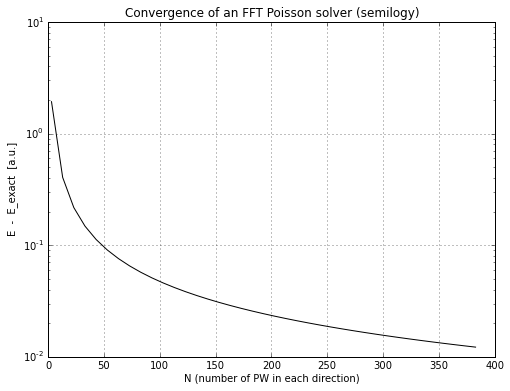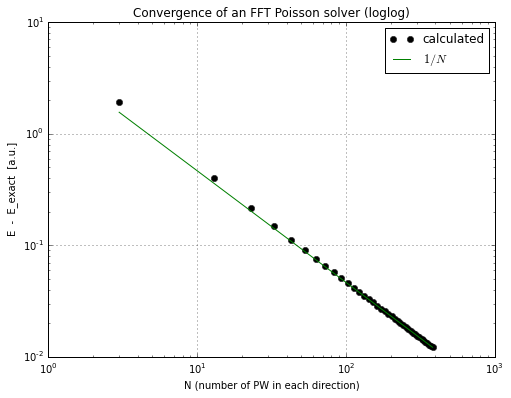In :
from pylab import plot, semilogy
N = D[:, 0]
E = D[:, 1]
E_exact = 128/(35*pi)

In :
figure(figsize=(8, 6), dpi=80)
semilogy(N, E-E_exact, "k-")
grid()
title("Convergence of an FFT Poisson solver (semilogy)")
xlabel("N (number of PW in each direction)")
ylabel("E  -  E_exact  [a.u.]")
savefig("fft_convergence_semilogy.png")In :
figure(figsize=(8, 6), dpi=80)
calculated = E-E_exact
loglog(N, E-E_exact, "ko", label="calculated")
predicted = 1/N
predicted = predicted * (calculated[-1] /  predicted[-1])
loglog(N, predicted, "g-", label="$1/N$")
grid()
title("Convergence of an FFT Poisson solver (loglog)")
xlabel("N (number of PW in each direction)")
ylabel("E  -  E_exact  [a.u.]")
legend()
savefig("fft_convergence_loglog.png")